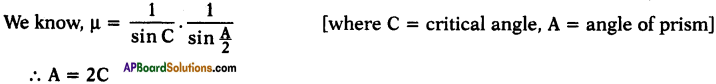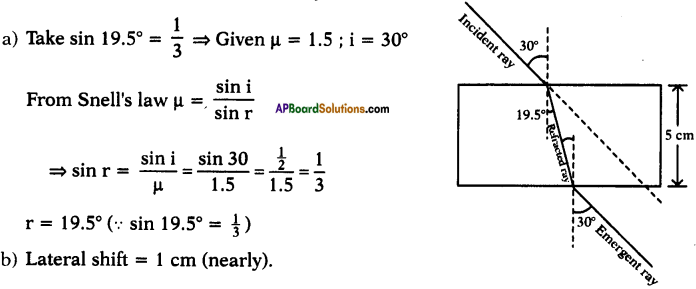# AP SSC 10th Class Physics Important Questions Chapter 5 Refraction of Light at Plane Surfaces

AP State Board Syllabus AP SSC 10th Class Physical Science Important Questions Chapter 5 Refraction of Light at Plane Surfaces.

## AP State Syllabus SSC 10th Class Physics Important Questions 5th Lesson Refraction of Light at Plane Surfaces

### 10th Class Physics 5th Lesson Refraction of Light at Plane Surfaces 1 Mark Important Questions and Answers

Question 1.
Take a bright metal ball and make it black with soot on a candle flame. Immerse it in the water. Mention one observation. (AP June 2015)
1) The ball shines.
2) The ball appears to raise up in water.

Question 2.
What is critical angle? (AP March 2015)
The angle of incidence at which the light ray propagates from denser to rarer graze along interface is called critical angle of denser medium.Question 3.
If refractive index of glass is $$\frac{3}{2}$$, then what is speed of light in glass? (AP June 2016)
(OR)
Find the speed of light in a transparent medium, whose refractive index is 3/2.
The refractive index of glass or transparent medium = $$\frac{3}{2}$$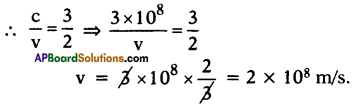Question 4.
Write any two questions about the ‘formation of mirages’. (AP June 2017)

1. When does a mirage form?
2. How does a mirage form?

Question 5.
Optical Fibre Cable (OFC) are oftenly used in tele-communications. What is the working principle behind the OFC? (AP March 2017)
Total Internal Reflection.

Question 6.
Among objects made of glass and diamond, which one shines more? Why? (AP June 2015)
Diamond shines more because of low conical angle of 24.4°.

Question 7.
Suggest reasons for the phenomenon associated with the following : Twinkling of stars. (TS March 2015)
Refraction of light is the reason for the twinkling of stars.

Question 8.
Draw the diagram showing the path of the ray when it travels from denser medium to rarer medium when the incident angle is more than the critical angle. (TS June 2016)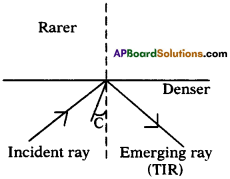Question 9.
Why does the light ray deviate in refraction? (AP SA-1:2019-20)
Light ray always chooses the path of least time to travel. Hence speed of light changes at interface of two media. So, the light ray deviate in refraction.

Question 10.
Name the phenomenon involved in the function of optical fibre. (AP SCERT : 2019-20)
Total Internal Reflection.

Question 11.
What is Fermat’s principle?
The light ray always travels in a path which needs shortest possible time to cover the distance between the two given points.

Question 12.
What happens when light travels from one medium to another medium?
It bends towards or away from normal.

Question 13.
When does speed of light decrease?
When it travels from rarer to denser medium.

Question 14.
What do you mean by denser medium?
The medium which has more optical density.

Question 15.
What is refraction?
The process of changing speed when light travels from one medium to another is called refraction of light.

Question 16.
Which quantity will compare the refractive indices of two media?
Relative refractive index.

Question 17.
What is relative refractive index?
It is the ratio of refractive index of second medium to refractive index of first medium.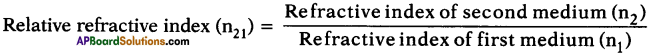Question 18.
When does a light ray bend away from normal?
When a light ray moves from denser to rarer medium it bends away from normal.

Question 19.
When will angle of refraction be equal to 90°?
When angle of incidence is equal to critical angle then angle of refraction will be equal to 90°.Question 20.
When angle of incidence of light ray is greater than critical angle, what happens?
Light ray undergoes total internal reflection.

Question 21.
What happens to refractive index of air with height?
Refractive index of air increases with height.

Question 22.
Which has greater refractive index between these?
1) cool air at the top
2) hotter air just above the road
Cooler air has greater refractive index due to more density.

Question 23.
What is mirage?
The virtual images of distant high objects cause the optical illusion called mirage.

Question 24.
What happens to a light ray when it falls perpendicular to one side of the slab surface?
It comes out without any duration.Question 25.
What are the conditions for total internal reflection?

1. The rays of light must travel from denser to rarer medium.
2. The angle of incidence of denser medium must be greater than critical angle.

Question 26.
What is meant by a vertical shift?
When a ray emerges out of a glass slab, it is parallel to the incident ray but is displaced laterally relative to incident ray. This shift of emergent ray is called vertical shift.

Question 27.
A ray of light travelling in air enters obliquely into water. Does the light ray bend towards the normal or away from the normal? Why?
It bends towards the normal. This is because it travels from an optically rarer to , optically denser medium.

Question 28.
When does Snell’s law fail?
Snell’s law fails when light is incident normally on the surface of a refracting medium.

Question 29.
Why does a ray of light bend when it travels into another medium?
It bends because its velocity changes when it moves from one medium to the other.

Question 30.
A pencil when dipped in a glass tumbler containing water appears to be bent at the interface of air and water. Explain why.

• When light travels obliquely from one transparent medium to another, the direction of propagation of light changes due to refraction of light.
• In this case, light travels from denser medium to rarer medium, hence it bends away from the normal and the pencil appears to be bent.

Question 31.
Why does light travel in vacuum?
Light travels in vacuum because it does not require medium for its propagation.Question 32.
What are the factors on which refractive index depends?

1. Nature of material
2. Wavelength of light used.

Question 33.
What does the ratio of sine of angle of incidence and sine of angle of refraction give?
The ratio of sine of angle of incidence and sine of angle of refraction gives refractive index.

Question 34.
What is the relationship between critical angle and refractive index?
The relationship between critical angle and refractive index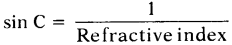Question 35.
What is the relationship between angle of incidence and shift?
As the angle of incidence increases, the shift also increases.Question 36.
Why does a coin placed in a water appear to be raised?
It is due to refraction of light.

Question 37.
Can you guess what happens when light travels from denser medium to rarer medium?
The light ray bends away from normal.

Question 38.
A ray of light falls normally on a face of a glass slab. What are the values of angle of incidence and angle of refraction of this ray?
Both angles are zero.Question 39.
When does light ray from slab not undergo any deviation?
The light ray that incidents perpendicular to one side of the slab surface comes out without any deviation.

Question 40.
What is the factor on which refraction depends?
Refraction depends on optical density.

Question 41.
What is absolute refractive index?
It is the ratio of speed of light in vacuum to speed of light in medium.
$$\mathrm{n}=\frac{\mathrm{c}}{\mathrm{v}}$$

Question 42.
In water filled vessel, the coin of the bottom can be seen at a height. Give reasons.
Rising of coin when water is poured in a cylindrical transparent vessel.

Question 43.
Write one activity in showing the process of ‘total internal refraction’.
Due to refraction of light speed of light changes when it travels from one medium to another medium.Question 44.
Define “Glass Slab”.
A thin glass slab is formed when a medium is isolated from its surroundings by two plane surfaces parallel to each other.

Question 45.
A ray of light is incident normally on a plane glass slab. What will be the angle of refraction and angle of deviation for the ray?
The ray is incident normally on a plane glass slab. So there is no deviation of light ray. Therefore the angle of refraction and angle of deviation both have 0° values.

Question 46.
A light ray in passing from water to a medium (a) speeds up, (b) slows down. In each case get one example of the medium.
a) Air, because its optical density is less than water,
b) Glass, because its optical density is more than water.

Question 47.
If an angle of refraction is 90°, what is the corresponding angle of incidence called?
The angle of incidence is called critical angle.Question 48.
If the angle of incidence is more than critical angle, what happens to light ray if the light ray travels from denser to rarer medium?
The light ray undergoes total internal reflection.

Question 49.
The refractive index of diamond is 2.42. What is the meaning of this statement in relation to speed of light?
It means that light travels 2.42 times faster in vacuum than in diamond.

Question 50.
For the same angle of incidence 45°, the angle of refraction in two transparent media, I and II is 20° and 30° respectively. Out of I and II, which medium is optically denser and why?
Medium I is optically dense as angle of refraction is lesser in it, hence light bends towards normal.

Question 51.
For which colour of white light is the refractive index maximum and for which colour of white light is the refractive index minimum?
The refractive index is maximum for violet because its wavelength is least.
The refractive index is minimum for red because its wavelength is maximum.

Question 52.
Correct the statement. “If the angle of incidence is greater than the critical angle the light is refracted when it falls on the surface from a denser medium to rarer medium”.
If the angle df incidence is greater than the critical angle the light undergoes total internal reflection when it falls on the surface from a denser medium to rarer medium.Question 53.
A light ray passes from medium 1 to medium 2. Which of the following quantities of refracted ray will differ from that of the incident ray?
Speed, intensity, frequency, wavelength.
Speed, intensity and wavelength will differ from that of incident ray.

Question 54.
The refractive indices of alcohol and turpentine oil with respect to air are 1.36 and 1.47 respectively. Find the refractive index of turpentine oil with respect to alcohol. Which one of these permits the light to travel faster?
The refractive index of turpentine oil with respect to alcohol = $$\frac{1.47}{1.36}$$ = 1.08.

The refractive index increases when the speed of light decreases. So light travels faster in alcohol as its refractive index is less.

Question 55.
Light enters from air to diamond which has refractive index of 2.42. Calculate the speed of light in diamond, if speed of light in air 3 × 108 ms-1.
Absolute refractive index = $$\frac{c}{v}$$
2.42 = $$\frac{3 \times 10^{8}}{\mathrm{v}}$$ ⇒ v = 1.24 × 108 ms-1.

Question 56.
A glass block 3.0 cm thick is placed over a stamp. Calculate the height through which image of stamp is raised. Refractive index of glass is 1.54.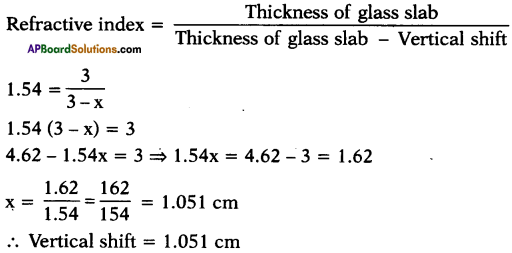Question 57.
The refractive index of water is 4/3. Calculate the critical angle for water – air interface (sin 49 = 3/4).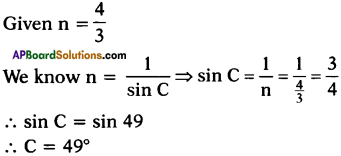### 10th Class Physics 5th Lesson Reflection of Light by Different Surfaces 2 Marks Important Questions and Answers

Question 1.
A ray of light enters from air to a medium X. The speed of light in the medium is 1.5 × 108 m/s and the speed of light in air is 3 × 108 m/s.
Find the refractive index of the medium X. (TS March 2015)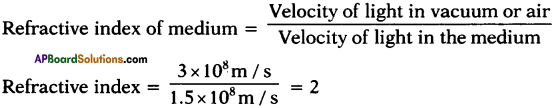Question 2.
What are the applications of optical fibres?
(OR)
Write two uses of fibre optics in daily life. (TS June 2016)
Applications oruses of optical fibres :

1. Light pipes using optical fibres may be used to see places which are difficult to reach things such as inside of a human body.
2. The other important application of fibre optics is to transmit communication signals through light pipes.

Question 3.
Focal length of the lens depends on its surrounding medium. What happens, if we use a liquid as surrounding media of refractive index, equal to the refractive index of lens? (TS June 2018)

• When the refractive index of surrounding media is equal to the refractive index of lens, the lens looses its characteristics.
• Lens do not diverge or converge the light.
• Light do not get refracted when it passes through that lens.Question 4.
Why does the light ray travel slowly in diamond when compared to vacuum? (AP SA-I : 2019-20)

• Refractive index of diamond (2.42) is greater than that of vacuum (1).
• Speed of light is inversely proportional to refractive index of substances.
• Hence, light ray travel slowly in diamond when compared to vacuum.

Question 5.
(OR)
Write the laws of refraction.
Laws of refraction :

1. The incident ray, the refractive ray and the normal to interface of two transparent media at a point of incidence lie in the same plane.
2. During refraction light follows Snell’s law, i.e., the ratio of sine of angled of incidence to sine of angle of refraction is constant.
n1 sin i = n2 sin r (OR) $$\frac{\sin \mathrm{i}}{\sin \mathrm{r}}$$ = constant.

Question 6.
What is total internal reflection ? What are the applications of total internal reflection?
When the angle of incidence is greater than critical angle, the light ray is reflected into denser medium at interface i.e., light never enters rarer medium. This phenomenon is called total internal reflection.
1) Brilliance of diamonds :
Total internal reflection is the main cause for brilliance of diamonds. The critical angle of diamonds is very low (24.4°). So if a light ray enters a diamond it is very likely to get total internal reflection which makes the diamond shine brilliant.

2) Optical fibres:
Total internal reflection is the basic principle for working of optical fibre.

Question 7.
What are optical fibres? How do they work?

• An optical fibre is very thin fibre made of glass or plastic having radius about a micrometer.
• A bunch of such thin fibres forms a light pipe.

Working :

1. Because of the small radius of the fibre, light going into it makes a nearly glancing incidence on the wall.
2. The angle of incidence is greater than the critical angle and hence total internal reflection takes place.
3. The light is thus transmitted along the fibre.

Question 8.
How can a patient’s stomach be viewed by using optical fibres?
(OR)
How do you observe patient’s stomach by using a light pipe?

• The patient’s stomach can be viewed by inserting one end of a light pipe into the stomach through the mouth.
• Light is sent down through one set of fibres in the pipe.
• This illuminates the inside of the stomach.
• The light from the inside travels back through another set of fibres in the pipe and the viewer gets the image at the outer end.

Question 9.
State four differences between reflection and total internal reflection.

 Reflection Total internal reflection 1) Smooth polished surface is required for reflection. 1) No smooth polished surface is required for total internal reflection. 2) It takes place for all angles of incidence. 2) It takes place only, when angle of incidence is greater than critical angle. 3) It takes place when the rays of light travel from rarer to denser medium to an opaque medium. 3) It takes place when rays of light travel from denser to rarer medium. 4) Some amount of light is absorbed by reflecting surface. 4) No light is absorbed by reflecting surface.

Question 10.
The figure shows refraction and emergence of a ray of light incident on a rectangular glass slab. Copy the diagram and mark the lateral displacement of the incident ray. Name the two factors on which the lateral displacement depends.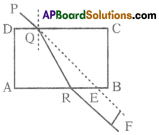The lateral displacement depends on

1. The angle of incidence of the incident ray PQ, on the slab and
2. The thickness of the glass slab.

The perpendicular distance between the emergent forward.

Question 11.
1) What happens to a ray of light when it travels from one equal refractive indices?
2) State the cause of refraction of light.
1) No refraction or bending would take place. The light will travel in a straight line.

2) The refraction occurs due to change in speed of light as it enters from one medium to another.Question 12.
A coin placed at the bottom of a tank appears to be raised when water is poured into it. Explain.

• It happens due to the phenomenon of refraction of light.
• When the rays of light from the coin, in the denser medium fall on the interface separating the two media, the rays of light move away from the normal after refraction.
• The point from which the refracted rays appear to come gives the apparent position of the coin.
• As the rays appear to come from a point above the coin, therefore, the coin seems to be raised.

Question 13.
Define refractive index. Explain the relationship between the refractive index of the medium and to the speed of light in the medium.
The ratio of speed of light in vacuum to the speed of light in that medium is defined as refractive index ‘n’ with respect to the vacuum. It is also called absolute refractive index.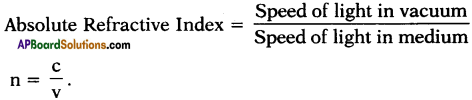When refractive index of a medium is high, then the speed of light is low and vice-versa.

Question 14.
Explain lateral shift and vertical shift.
Lateral shift:
The distance between incident and emergent ray is called lateral shift.

Vertical shift :
The perpendicular distance between object and its image is called vertical shift.

Question 15.
During refraction of light, which of the following quantities does not change.
(1) velocity,
(2) wavelength,
(3) frequency,
(4) amplitude.
During refraction of light velocity of light changes and also wavelength and amplitude. Frequency does not change during refraction.

Question 16.
The upper surface of water contained in a beaker and held above the eye level appears silvery. Why?
Critical angle for water is 48°. The rays of light entering in water from below, suffer refraction. If these rays strike the water-air surface at an angle which is greater than 48°, they get totally internally reflected. These rays on emerging out of water, appear to come from the upper surface of water, which in turn appear silvery.

Question 17.
Why don’t the planets twinkle?

• The planets are much closer to the earth, and are thus seen as extended sources.
• We can consider a planet as a collection of a large number of point-sized sources of light.
• The total variation in the amount of light entering our eye from all the individual point-sized sources will average out to zero, thereby nullifying twinkling effect

Question 18.
Why did an empty test tube placed obliquely in water, appears filled with mercury, when seen from above?
When the rays of light travelling through water they strike the water glass interface of test tube at an angle, which is more than critical angle for water, they suffer total internal reflection. When these totally reflected rays reach eye, then to the eye they appear as they come from surface of test tube, which in turn appears filled with mercury.Question 19.
Why are the bubbles rising up the fish tank appear silvery?
When the rays of light travelling through water they strike the water air interface of the bubble at an angle, which is greater than critical angle for water, they get totally internally reflected. These reflected rays on reaching the eye appear to come from air bubble, which in turn appears silvery.

Question 20.
Why does a crack in a window pane appear silvery?
There is always some amount of air present in the crack. When the rays of light travelling through glass, strike the glass, the glass air interface at an angle, greater than critical angle of glass, they are totally internally reflected. When these reflected rays reach eye, then to the eye they appear to come from the crack, which in turn appears silvery.

Question 21.
Explain why a straight stick appears to be bent when dipped in water.

• Suppose two rays originate from the end of the stick in water.
• As these rays get refracted into the air, they bend away from the normal.
• When these two refracted rays are produced backwards they seem to meet at a point higher than the end of stick.
• This point gives the apparent position of the end of the stick. Thus, the stick appears to be bent.

Question 22.
A pond appears to be shallower than it really is when viewed obliquely. Why?

• Suppose two rays originate from the bottom of the pond. As these rays get refracted into the air, they bend away from the normal.
• When these two refracted rays are produced backwards they seem to meet at a point higher than the bottom of the pond.
• This point gives the apparent position of the bottom of the pond.
• Thus, the pond appears to be shallower.
• This effect is absent if the pond is viewed normally.

Question 23.
Frame some questions to know about the formation of mirages.

1. What are mirages?
2. What is the principle involved in mirages?
3. Can mirages be photographed?
4. Where does the water on the road go?Question 24.
A glass slab is placed over a piece of paper on which VIBGYOR is printed with each letter into corresponding colours.
1) Will the image of all the letters be in the same place?
2) The letter of which colour appears to be raised maximum and which colour minimum? Explain your answer.

1. The image of all letters will not be in the same place.
2. The letter of violet colour appears to be raised maximum, while the letter of red colour appears to be raised minimum because refractive index of glass is most for the violet light while least for the red light, therefore the apparent depth is least for violet and most for red.

Question 25.
Why does sun appear bigger during the sunset or the sunrise?

1. We already know that the apparent position of sun is higher than actual position in the horizon.
2. Moreover, due to refraction, the apparent image of sun is closer to eye than the actual position. Since during sunset or sunrise, the rays of light travel through maximum length of atmosphere therefore the refraction is also maximum.
3. Hence apparent image of sun is very much closer to eye. Thus it appears bigger.

Question 26.
Write the material required in finding out the relation between angle of incidence and angle of refraction.
Material required :
A plank, white chart, protractor, scale, small black painted plank, a semi-circular glass disc of thickness nearly 2cm, pencil and laser light.

Question 27.
Write the aim and apparatus experiment in finding the refractive index of the glass slab.
Aim :
Finding the refractive index of the glass slab.

Apparatus :
Glass slab, white chart, pin.

Question 28.
Observe the following table and answer the following question.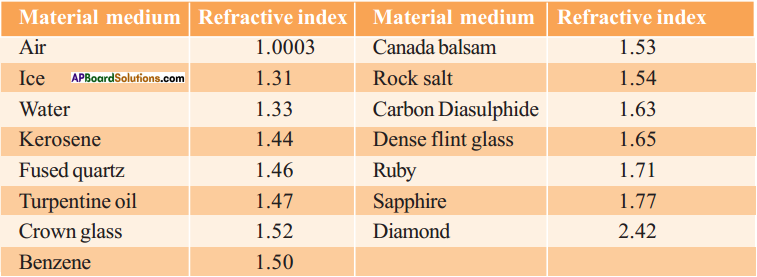Questions :
1) Find out from the table the medium having highest optical density and the medium with lowest optical density.
2) You are given kerosene, turpentine oil and water. In which of these does the light travel fast? Use the information given in the table.
3) The refractive index of diamond is 2.42. What is the meaning of this statement?
4) When light travels from water to crown glass, what happens?
5) When light travels from diamond to air, what happens?
1) The medium with highest optical density is diamond as its refractive index is maximum, i.e. 2.42.
The medium with lowest optical density is air, as its refractive index is minimum, i.e. 1.0003.

2) The refractive index of medium is given by the expression, n = $$\frac{c}{v}$$ or v = $$\frac{c}{n}$$
This expression shows that light travels faster in the medium whose refractive index is minimum. From the table, we can find that water has the minimum value of refractive index. Therefore light travels faster in water.

3) This statement means that light travels 2.42 times faster in vacuum than in diamond.

4) The light bends towards normal.

5) The light bends away from the normal.

Question 29.
A ray of light enters from a medium A into a slab made up of a transparent substance B. Refractive indices of medium A and B are 2.42 and 1.65 respectively. Complete the path of ray of light till it emerges out of slab.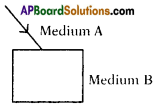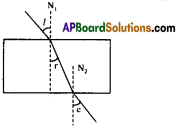Question 30.
A glass slab made of material of refractive index n1 is kept in medium of refractive index n2. A light ray is incident on the slab. Complete the path of rays of light emerging from glass slab, if a) n1 > n2 b) n1 = n2 c) n1 < n2.
a) n1 > n2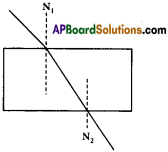b) n1 = n2
There is no deviation of light ray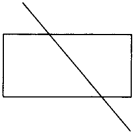c) n1 < n2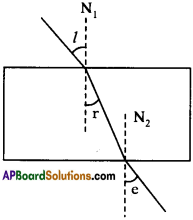Question 31.
How do you appreciate the process of total internal reflection in nature?

1. Total internal reflection is responsible for brilliance of diamond.
2. Total internal reflection is basic pruxiplo behind working of optical fibres which are used in getting the images ol internal ouaiis and also used in telecommunications. So the role of total internal reflection is thoroughly appreciated.

Question 32.
Write the application of optical fibres in communication.

• Optical fibres are used to transmit communication signals through light pipes.
• For example, about 2000 telephone signals, approximately mixed with lightwaves, may be simultaneously transmitted through a typical optical fibre.
• The clarity of the signals transmitted in this way is much better than other conventional methods.Question 33.
Write the applications of total internal reflection.
Application of total internal reflection :

1. Brilliance of diamonds,
2. Optical fibres.

Question 34.
A monochromatic ray of light strikes the surface of transparent medium at an angle of incidence 60° and gets refracted into the medium at an angle of refraction 45°. What is the refractive index of the medium?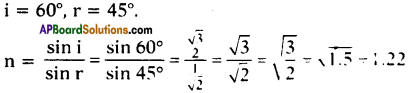Question 35.
A light ray enters a liquid at an angle of incidence 45° and it gets refracted on liquid at angle of refraction 30°. Calculate the refractive index of the liquid.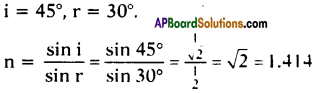Question 36.
Refractive index of water is 4/3. Calculate the speed of light in water.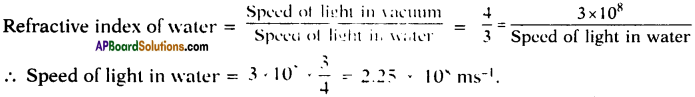Question 37.
A postage stamp placed under glass appears raised by 8 mm. If refractive index of glass is 1.5, calculate the actual thickness of glass slab.
Let real thickness of glass = x.
Vertical shift = 8 mm.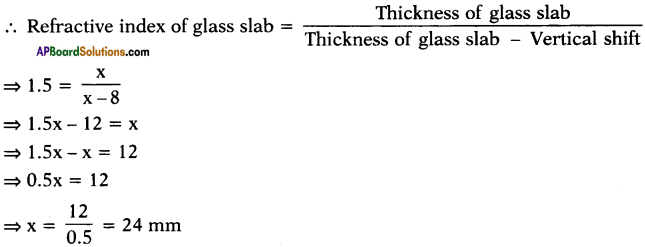Question 38.
Refractive index of glass is 1.5. Find its critical angle.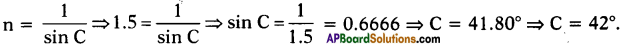Question 39.
What is the advantage of using prism in place of plane mirror in periscope or binocular?

• When total internal reflection occurs from a prism, the entire incident light is reflected back into the denser medium.
• Whereas in ordinary reflection from a plane mirror, some light is refracted and absorbed. So the reflection is partial.
• This is the reasons why total reflecting prism is used in place of a plane mirror to deviate the light ray by 90° in a periscope and 180° in a binocular.

### 10th Class Physics 5th Lesson Reflection of Light by Different Surfaces 4 Marks Important Questions and Answers

Question 1.
What is the angle of deviation produced by the glass slab? Explain with ray diagram. (AP June 2015)
(OR)
Which angle of deviation is produced by glass slab? Write your explanation with a ray diagram.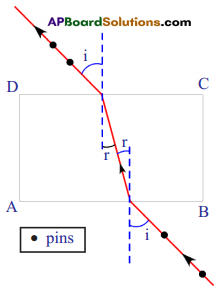1. Angle of deviation is the angle between incident ray and emergent ray.
2. The angle of deviation produced by a glass slab is ‘O’, because the incident ray and emergent ray are parallel to each other that can be seen in the figure.

Question 2.
Explain the phenomenon of total internal reflection with two examples. (AP June 2018)
(OR)
What is total internal reflection? Explain with examples. (AP SA-I:2019-20)

• When the angle of incidence is greater than the critical angle, the light ray is reflected into denser medium at interface. This phenomenon is called total internal reflection.
• Total internal reflection is the main reason for brilliance of diamonds. The critical angle of a diamond is very low. So if a light ray enters a diamond it is very likely to undergo total internal reflection which makes the diamond shine.
• Total internal reflection is the basic principle behind working of optical fibre. Because of the small radius of the fibre light going into it makes a nearly glancing incidence is greater than the critical angle and hence total internal reflection takes place. The light is thus transmitted along the fibre.

Question 3.
Explain the relation between angle of incidence and angle of refraction with an experiment. (AP March 2018)
Aim :
To verify the relation between angle of incidence and angle of refraction.

Material required :
A plank, white chart, protractor, semicircular glass disc, pencil and leser light.

Procedure :

1. Take a drawing sheet on a cardboard and mark different angles (on both side of MM line)
2. Place a semi circular glass disc, so that its diameter coincides with the line “MM”.
3. Send a laser light along a line with makes 15° with NN.
4. Let it is incident angle.
5. Measure its corresponding angle of refraction by observing light coming from outside of the glass slab.
6. Repeat this experiment with various values of angle of incidence, refraction and not in the table.

7.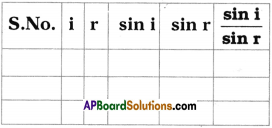8. From the above table we observe that $$\frac{\sin \mathrm{i}}{\sin r}$$ = constant.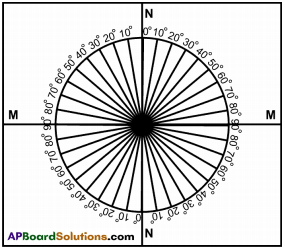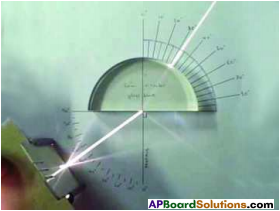Question 4.
Give some daily life consequences of refraction of light.

• A star appears twinkling in the sky.
• The sun is seen a few minutes before it rises above the horizon in the morning and in the evening few minutes longer after it sets.
• A coin kept in a vessel not visible when seen from just below the edge of the vessel, can be viewed from the same position when water is poured into the vessel.
• A print appears to be raised when a glass block placed over it.
• A piece of paper stuck at the bottom of a glass block appears to be raised when seen from above.
• A tank appears shallow than its actual depth.
• A person’s legs appear to be short when standing in a tank.
• An object placed in a denser medium when viewed from a rarer medium appears to be at a lesser depth.
• An object in a rarer medium, when viewed from a denser medium, appears to be at a greater distance than its real distance.Question 5.
What are the factors which influence refractive index of material?

• Nature of medium, i.e. its optical density. Smaller the speed of light in a medium relative to air, higher is the refractive index of the medium.
• Physical condition such as temperature. With rise in temperature the speed of light in medium increases, so the refractive index of medium decreases.
• The colour or wavelength of light (refractive index increases with decrease in wavelength, eg : µv > µR).

Question 6.
What is the advantage of total internal reflection over reflection?

• In the process of total internal reflection, 100% energy is reflected back.
• No other device such as plane mirror, etc. produces 100% reflection due to absorption and refraction of some part of light.
• Due to this property the phenomenon total internal reflection is of great practical application in the construction of periscope, binocular and certain type of camera.

Question 7.
The diagram below shows a glass block suspended in a liquid. A beam of light of single colour is incident from liquid on one side of block.
1) Draw diagrams to show how light bends when it travels from liquid to glass and then to liquid if (i) the light slows down in glass (ii) the light speeds up in glass.
2) State two conditions under which the light ray moving from liquid to glass passes straight without bending. Will the glass be visible them?
1) If light slows down in going from liquid to glass (i.e., µglass > µliquid), it will bend towards the normal at the point of incidence in passing from liquid to glass at the first surface, while it is bent away from normal at the second surface in passing from glass to liquid. In the ray diagram, the light beam suffers lateral shift.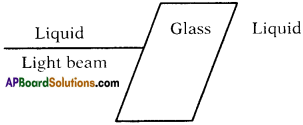2) If light speeds up in going from liquid to glass (i.e., µglass < µliquid). It will bend away from the normal at the point of incidence on the first surface in passing from liquid to glass, while it bends towards the normal at the second surface in passing from glass to liquid. The light beam suffers lateral shift in direction opposite to that
Note that in both cases, the emergent ray is parallel to the incident ray.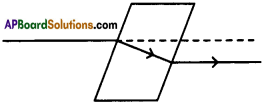Question 8.
A ray of light is incident on a rectangular glass block PQRS, which is silvered at the surface RS. The ray is partly reflected and partly refracted.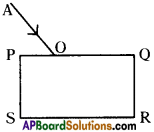1) Trace the path of reflected and refracted rays.
2) Show at least two rays emerging from the surface PQ after reflection from the surface RS.
3) How many images are formed in the above case? Which image is the brightest?
1) In the figure OB is reflected ray and OC is the refracted ray for the incident ray AO.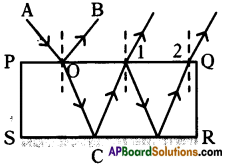2) Two rays emerging from surface PQ after reflections for the surface RS are labelled as 1 and 2.

3) Multiple (or infinite) images are formed. The second image formed due to first reflection at C at the silvered surface RS is the brightest. It is seen in the direction of ray 1.

Question 9.
What are the factors which affect critical angle? The critical angle for a given pair of media depends on their refractive index which is affected by the following factors.
1. Effect of colour of light:
The refractive index of transparent medium is more for violet light and less for red light, therefore the critical angle for pair of media is less for the violet light and more for the red light. Thus critical angle increases with increase in wavelength of light.

2. Effect of temperature :
On increasing the temperature of medium, its refractive index decreases, so the critical angle for that pair of media increases. Thus critical angle increases with increase in temperature.

Question 10.
The table shows the refractive index of some material media.

 Material Medium Refractive Index Air 1.0003 Ice 1.31 Water 1.33 Kerosene 1.44 Fused quartz 1.46 Turpentine oil 1.47 Crown glass 1.52 Benzene 1.50

Answer the following questions with the help of the above table.
1) Find the speed of light in Benzene.
2) Write the relationship between mass density and optical density of kerosene and water.
3) What are the factors that refractive index depends on?
4) Write the relative refractive index of kerosene with water.
1)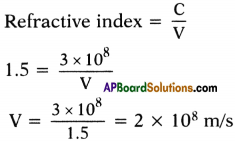Speed of light in benzene = 2 × 108 m/s

2) Optical denser medium may not possess greater mass density. Kerosene with high refractive index is optically denser than water although its mass density is lesser than water.

3) Refractive index depends on
1) nature of material,
2) wavelength of light used.

4) Relative refractive index of kerosene with water = $$\frac{1.44}{1.33}$$ = 1.08

Question 11.
Red light of wavelength 6600A travelling in air gets refracted in water. If the speed of light in air is 3 × 108 ms-1 and refractive index of water is 4/3, find the
(i) frequency of light in air,
(ii) the speed of light in water,
(iii) the wavelength of light in water.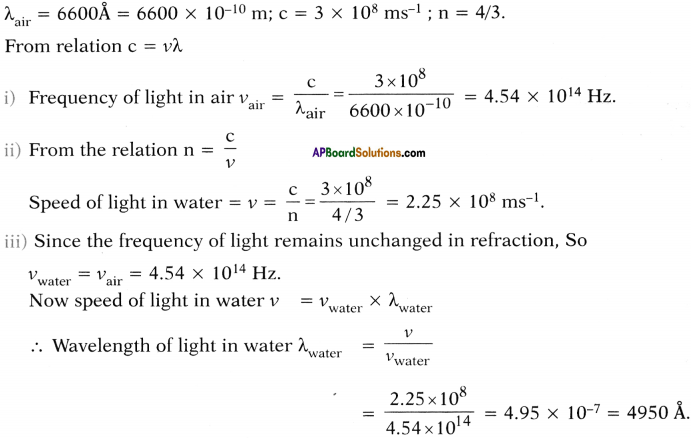Question 12.
Draw the ray diagram which shows the ray takes curved path because of total internal reflection.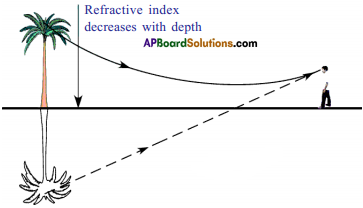Question 13.
Give some daily life consequences of total internal reflection?

• On a hot sunny day, a driver may see a pool of water on the road before him. It is the phenomenon of mirage which is often observed in desert.
• An empty test tube placed in a beaker with mouth outside the water surface shines like a mirror.
• A crack in a glass vessel often shines like a mirror.
• A piece of diamond sparkles when viewed from certain directions.
• An optical fibre is used to transmit a light signal over a long distance with negligible loss of energy.Question 14.
Light travels from air to water, then the refraction index of water is 1.33. Hence find the refractive index when light travels from water to air.
Refractive index of water (n21) = 1.33
Refractive index of air (n12) = $$\frac{1}{1.33}$$ = 0.75

Question 15.
The refractive index of diamond is 2.42 and the refractive index of glass is 1.5; compare the critical angle between them. (Diamond 24°, glass 42°)
Refractive index of diamond (µ1) = 2.42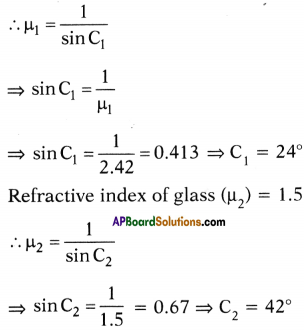Question 16.
A ray of light travels from an optically denser to rarer medium. The critical angle of the two media is ‘C’. What is the maximum possible deviation of the ray?